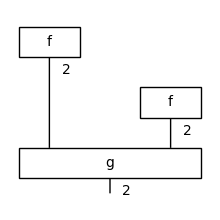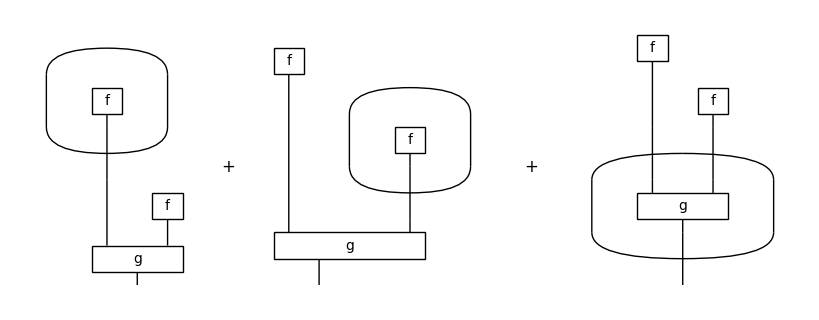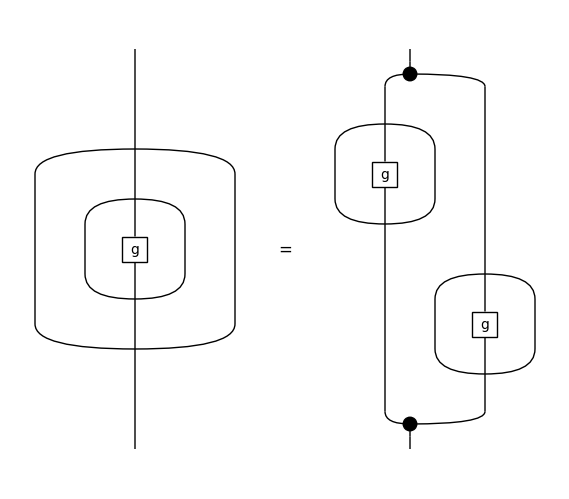# Diagrammatic Differentiation#

Quantum Group Workshop, 26th January 2021

Alexis Toumi, joint work with Richie Yeung and Giovanni de Felice

https://doi.org/10.4204/EPTCS.343.7

## 1) Parametrised matrices#

Fix a rig $$(\mathbb{S}, +, \times, 0, 1)$$.

Definition: A matrix $$f \in \mathbf{Mat}_\mathbb{S}(m, n)$$ is a function $$f : [m] \times [n] \to \mathbb{S}$$ where $$[n] = \{0, \dots, n - 1\}$$ for $$n \in \mathbb{N}$$.

Definition: A parametrised matrix is a function $$f : \mathbb{S} \to \mathbf{Mat}_\mathbb{S}(m, n)$$, or equivalently a function-valued matrix $$f \in \mathbf{Mat}_{\mathbb{S} \to \mathbb{S}}(m, n)$$.

Example:

:

from discopy.quantum.gates import Rz
from sympy import Expr
from sympy.abc import phi

Rz(phi).array

:

array([[exp(-1.0*I*pi*phi), 0],
[0, exp(1.0*I*pi*phi)]], dtype=object)

:

(lambda phi: Rz(phi).array)(0.25)

:

array([[0.70710678-0.70710678j, 0.        +0.j        ],
[0.        +0.j        , 0.70710678+0.70710678j]])


## 2) Parametrised diagrams#

Fix a monoidal signature $$(\Sigma_0, \Sigma_1, \text{dom}, \text{cod} : \Sigma_1 \to \Sigma_0^\star)$$ for $$X^\star = \coprod_{n \in \mathbb{N}} X^n$$ the free monoid.

Definition: An abstract diagram $$d \in \mathbf{C}_\Sigma(s, t)$$ is defined by a list of $$\text{layers}(d) = (\text{left}_0, \text{box}_0, \text{right}_0), \dots, (\text{left}_n, \text{box}_n, \text{right}_n) \in \Sigma_0^\star \times \Sigma_1 \times \Sigma_0^\star$$.

Definition: A concrete diagram is an abstract diagram with a monoidal functor $$F : \mathbf{C}_\Sigma \to \mathbf{Mat}_\mathbb{S}$$.

Definition: A parametrised diagram is a function $$d : \mathbb{S} \to \mathbf{C}_\Sigma(s, t)$$, or equivalently, a diagram with a monoidal functor $$F : \mathbf{C}_\Sigma \to \mathbf{Mat}_{\mathbb{S} \to \mathbb{S}}$$.

Example:

:

from discopy.tensor import Dim, Box

x, y, z = Dim(1), Dim(2), Dim(2)
f = Box('f', x, y, [phi + 1, -phi * 2])
g = Box('g', y @ y, z, [1, 0, 0, 0, 0, 0, 0, phi ** 2 + 1])

d = f @ f >> g
print(d)
d.draw(figsize=(2, 2))

d.eval(dtype=Expr).array

f >> Dim(2) @ f >> g:

array([(phi + 1)**2, 4*phi**2*(phi**2 + 1)], dtype=object)

:

d.subs(phi, 0.25).eval(dtype=Expr).array

:

array([1.5625, 0.265625], dtype=object)


## 3) Product rule#

We define the gradient of a parametrised matrix $$f \in \mathbf{Mat}_{\mathbb{S} \to \mathbb{S}}(m, n)$$ elementwise:

$\frac{\partial f}{\partial x}(i, j) = \frac{\partial}{\partial x} f(i, j)$

Given a parametrised diagram $$d, F$$ we want a new diagram $$\frac{\partial d}{\partial x}$$ such that:

$F\big(\frac{\partial d}{\partial x}\big) = \frac{\partial F(d)}{\partial x}$

We can do this by defining gradients as formal sums of diagrams and using the product rule:

$\frac{\partial}{\partial x} (d \otimes d') = \frac{\partial d}{\partial x} \otimes d' + d \otimes \frac{\partial d'}{\partial x}$
$\frac{\partial}{\partial x} (d \circ d') = \frac{\partial d}{\partial x} \circ d' + d \circ \frac{\partial d'}{\partial x}$

Example:

:

d.grad(phi).draw(figsize=(8, 3), draw_type_labels=False)## 4) Chain rule#

Given an arbitrary function $$f : \mathbb{S} \to \mathbb{S}$$, we lift it to matrices by applying it elementwise. Diagrammatically, we represent this as a bubble around a subdiagram.

Gradients of bubbles are then given by the chain rule:

$\frac{\partial}{\partial x} f(d) = \frac{\partial f}{\partial x} (d) \times \frac{\partial d}{\partial x}$

where the elementwise product can be encoded as pre- and post-composition with spiders.

:

g = Box('g', Dim(2), Dim(2), [2 * phi, 0, 0, phi + 1])
f = lambda d: d.bubble(func=lambda x: x ** 2, drawing_name="f")